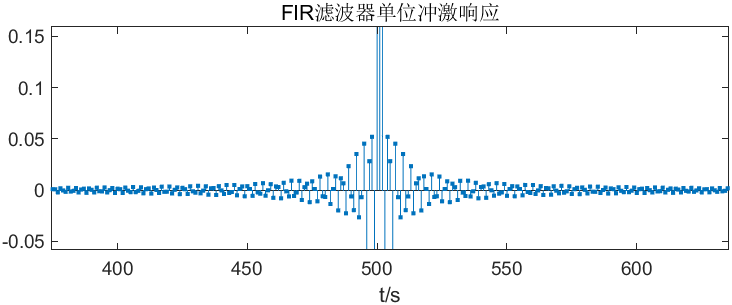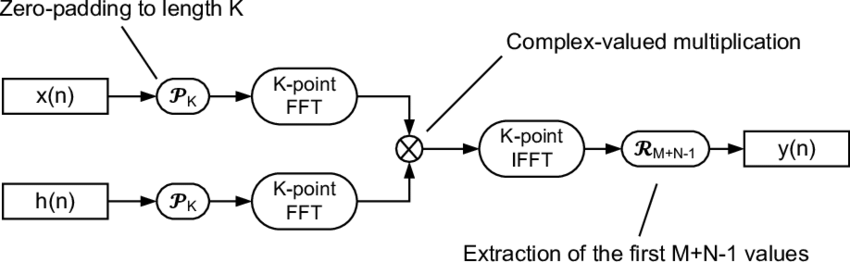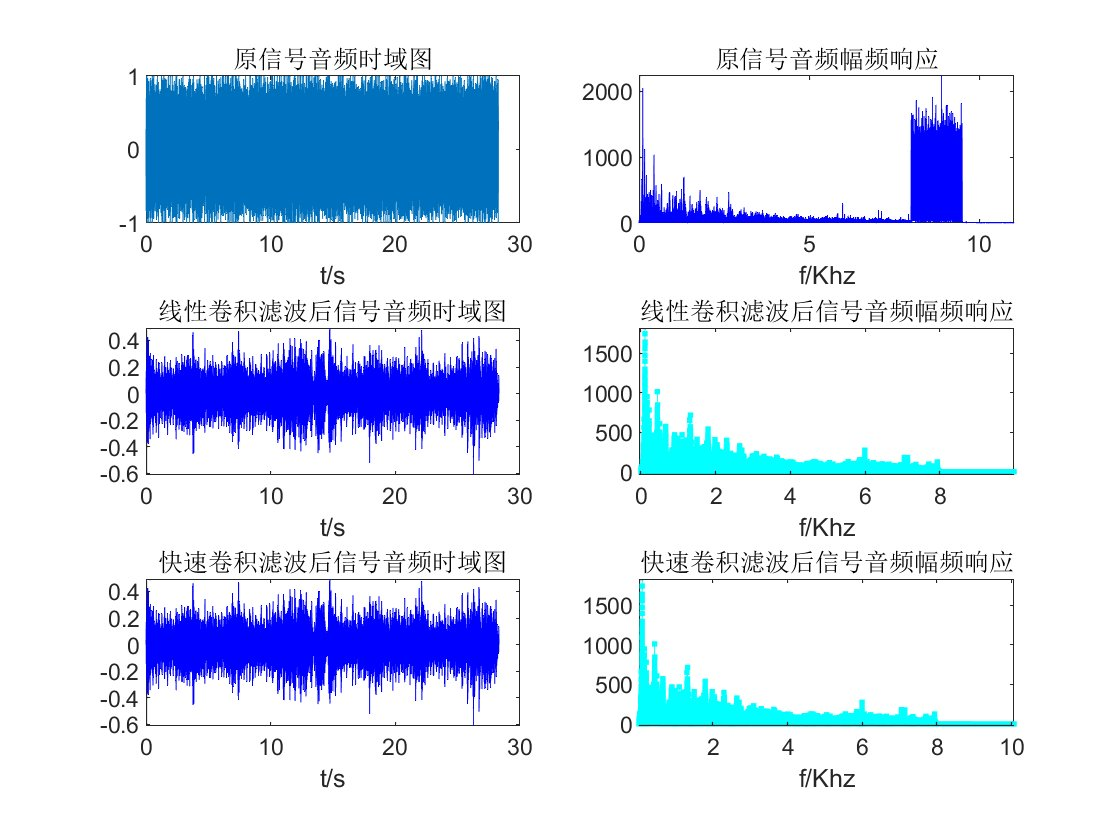# FIR滤波器结合快速卷积滤波

## 二、实现思路

### 设计FIR滤波器

• 分析原始音频信号的频谱，观察有用信号和噪声的分布，选择滤波器类型（低通、高通、带通、带阻等）
• 设定幅频响应下降三分贝的截止频率和滤波器阶数
• 选择合适的窗函数（包括Rectangular、Hanning、Hamming、Bartlett、Blackman、Kaiser、Gaussian、Flat-top等）
• 获得滤波器的单位采样响应hn

### 快速卷积

• 计算原始音频信号x与滤波器的单位采样响应hn的线性卷积长度，L=length(x)+length(hn)
• 设计FFT的长度N>=L
• 对x和hn分别做N点FFT变换获得Xk、Hk
• 将Xk与Hk相乘得到Yk
• 将Yk做IFFT逆变换得到yn，即完成快速卷积

## 三、实现过程

### FIR滤波器

H ( z ) = ∑ k = 0 N − 1 h ( k ) ∗ z − k H(\mathrm{z})=\sum_{k=0}^{N-1}h(\mathrm{k})*\mathrm{z}^{-k}

y ( n ) = ∑ m = 0 N − 1 h ( m ) x ( n − m ) \mathrm{y(n)}=\sum_{m=0}^{N-1}\mathrm{h(m)x(n-m)}

%% hamming窗低通滤波器设计
fc=7.95e3; %下降3分贝截止频率
hn=fir1(1000,fc*2/Fs,"low");
hn=hn';
Nh=length(hn);


FIR滤波器的单位冲击响应如下图所示：### 快速卷积%% 快速卷积和线性卷积
L=Nx+Nh-1;
Xk=fft(x,L);%计算x的L点FFT,结果为X(k)
Hk=fft(hn,L);%计算hn的L点FFT,结果为H(k)
Yk=Xk.*Hk;%计算YK
y1n=ifft(Yk,L);%对YK调用IFFT，求得y1(n)
y2n=conv(x,hn);%计算y2(n)的卷积


## 四、结果展示

### 线性卷积和FFT快速卷积滤波前后对比图## 五、结果分析

• 由上图可知普通线性卷积的结果和快速卷积的结果一样，但快速卷积大大提高了运算速率，减少了运算量，提高了运算效率。
• 离散傅立叶变换（DFT)实现了频域的离散化,方便了计算机处理,在数字信号处理中有着非常重要的作用。但直接计算DFT的运算量与变换长度N的平方成正比,计算量太大。而快速傅立叶变换FFT则是快速计算DFT的有效算法，大大提高了DFT的运算效率，在信号频谱的分析、滤波器频率响应的计算，以及线性卷积的快速计算等方面起着非常重要的作用。

## 代码开源

### 网站公告